Search by Topic

Resources tagged with Networks/Graph Theory similar to Magic Squares II:

Filter by: Content type:
Age range:
Challenge level:

There are 29 results

Broad Topics > Decision Mathematics and Combinatorics > Networks/Graph TheorySprouts Explained

Age 7 to 18

This article invites you to get familiar with a strategic game called "sprouts". The game is simple enough for younger children to understand, and has also provided experienced mathematicians with. . . .Geometry and Gravity 2

Age 11 to 18

This is the second of two articles and discusses problems relating to the curvature of space, shortest distances on surfaces, triangulations of surfaces and representation by graphs.Some Circuits in Graph or Network Theory

Age 14 to 18

Eulerian and Hamiltonian circuits are defined with some simple examples and a couple of puzzles to illustrate Hamiltonian circuits.Knight Defeated

Age 14 to 16 Challenge Level:

The knight's move on a chess board is 2 steps in one direction and one step in the other direction. Prove that a knight cannot visit every square on the board once and only (a tour) on a 2 by n board. . . .Classifying Solids Using Angle Deficiency

Age 11 to 16 Challenge Level:

Toni Beardon has chosen this article introducing a rich area for practical exploration and discovery in 3D geometryDice, Routes and Pathways

Age 5 to 14

This article for teachers discusses examples of problems in which there is no obvious method but in which children can be encouraged to think deeply about the context and extend their ability to. . . .Plum Tree

Age 14 to 18 Challenge Level:

Label this plum tree graph to make it totally magic!Pattern of Islands

Age 11 to 14 Challenge Level:

In how many distinct ways can six islands be joined by bridges so that each island can be reached from every other island...Symmetric Tangles

Age 14 to 16

The tangles created by the twists and turns of the Conway rope trick are surprisingly symmetrical. Here's why!Königsberg

Age 11 to 14 Challenge Level:

Can you cross each of the seven bridges that join the north and south of the river to the two islands, once and once only, without retracing your steps?Konigsberg Plus

Age 11 to 14 Challenge Level:

Euler discussed whether or not it was possible to stroll around Koenigsberg crossing each of its seven bridges exactly once. Experiment with different numbers of islands and bridges.Tourism

Age 11 to 14 Challenge Level:

If you can copy a network without lifting your pen off the paper and without drawing any line twice, then it is traversable. Decide which of these diagrams are traversable.Magic Caterpillars

Age 14 to 18 Challenge Level:

Label the joints and legs of these graph theory caterpillars so that the vertex sums are all equal.Magic W

Age 14 to 16 Challenge Level:

Find all the ways of placing the numbers 1 to 9 on a W shape, with 3 numbers on each leg, so that each set of 3 numbers has the same total.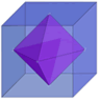The Four Colour Theorem

Age 11 to 16

The Four Colour Conjecture was first stated just over 150 years ago, and finally proved conclusively in 1976. It is an outstanding example of how old ideas can be combined with new discoveries. prove. . . .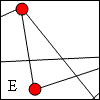Travelling Salesman

Age 11 to 14 Challenge Level:

A Hamiltonian circuit is a continuous path in a graph that passes through each of the vertices exactly once and returns to the start. How many Hamiltonian circuits can you find in these graphs?Tangles

Age 11 to 16

A personal investigation of Conway's Rational Tangles. What were the interesting questions that needed to be asked, and where did they lead?Round-robin Scheduling

Age 7 to 14 Challenge Level:

Think about the mathematics of round robin scheduling.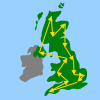The Olympic Torch Tour

Age 14 to 16 Challenge Level:

Imagine you had to plan the tour for the Olympic Torch. Is there an efficient way of choosing the shortest possible route?Factors and Multiples Graphs

Age 14 to 18 Challenge Level:

Explore creating 'factors and multiples' graphs such that no lines joining the numbers cross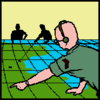Going Places with Mathematicians

Age 7 to 14

This article looks at the importance in mathematics of representing places and spaces mathematics. Many famous mathematicians have spent time working on problems that involve moving and mapping. . . .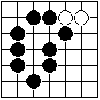Behind the Rules of Go

Age 14 to 18

This article explains the use of the idea of connectedness in networks, in two different ways, to bring into focus the basics of the game of Go, namely capture and territory.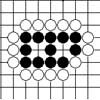Sufficient but Not Necessary: Two Eyes and Seki in Go

Age 14 to 18

The game of go has a simple mechanism. This discussion of the principle of two eyes in go has shown that the game does not depend on equally clear-cut concepts.Instant Insanity

Age 11 to 18 Challenge Level:

Given the nets of 4 cubes with the faces coloured in 4 colours, build a tower so that on each vertical wall no colour is repeated, that is all 4 colours appear.Ding Dong Bell

Age 11 to 18

The reader is invited to investigate changes (or permutations) in the ringing of church bells, illustrated by braid diagrams showing the order in which the bells are rung.Fermat's Poser

Age 14 to 16 Challenge Level:

Find the point whose sum of distances from the vertices (corners) of a given triangle is a minimum.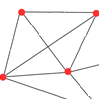Only Connect

Age 11 to 14 Challenge Level:

The graph represents a salesman’s area of activity with the shops that the salesman must visit each day. What route around the shops has the minimum total distance?Olympic Magic

Age 14 to 16 Challenge Level:

in how many ways can you place the numbers 1, 2, 3 … 9 in the nine regions of the Olympic Emblem (5 overlapping circles) so that the amount in each ring is the same?A Curious Collection of Bridges

Age 11 to 18

Read about the problem that tickled Euler's curiosity and led to a new branch of mathematics!